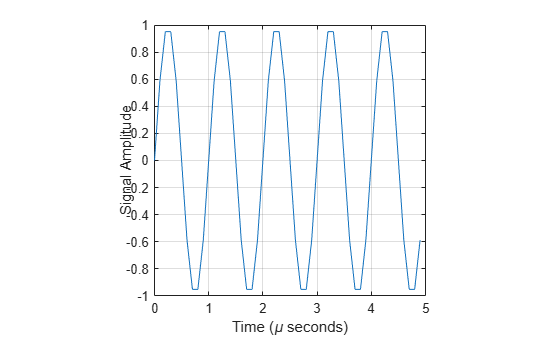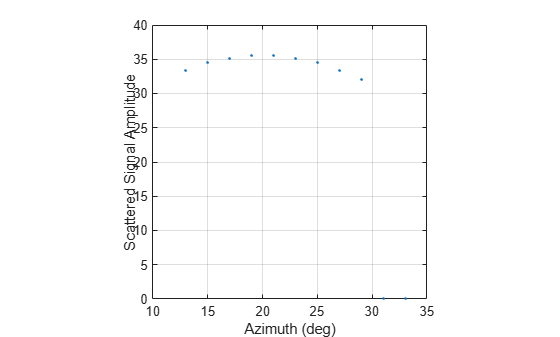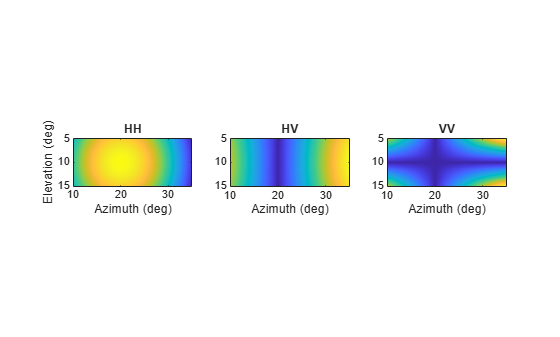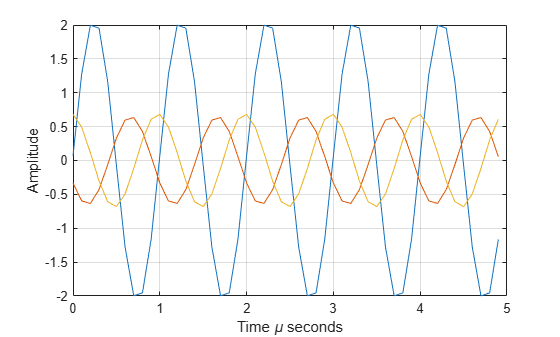# step

Package: phased

Backscatter incoming signal

## Syntax

refl_sig = step(target,sig,ang)
refl_sig = step(target,sig,ang,update)
refl_sig = step(target,sig,ang,laxes)
refl_sig = step(target,sig,ang,laxes,update)

## Description

Note

Starting in R2016b, instead of using the step method to perform the operation defined by the System object™, you can call the object with arguments, as if it were a function. For example, y = step(obj,x) and y = obj(x) perform equivalent operations.

example

refl_sig = step(target,sig,ang) returns the reflected signal, refl_sig, of an incident nonpolarized signal, sig, arriving at the target from the angle, ang. This syntax applies when you set the EnablePolarization property to false and the Model property to 'Nonfluctuating'. In this case, the values specified in the RCSPattern property are used to compute the RCS values for the incident and reflected directions, ang.
refl_sig = step(target,sig,ang,update) uses update to control whether to update the RCS values. This syntax applies when you set the EnablePolarization property to false and the Model property to one of the fluctuating RCS models: 'Swerling1', 'Swerling2', 'Swerling3', or 'Swerling4'. If update is true, a new RCS value is generated. If update is false, the previous RCS value is used.
 refl_sig = step(target,sig,ang,laxes) returns the reflected signal, refl_sig, of an incident polarized signal, sig. The matrix, laxes, specifies the local target coordinate system. This syntax applies when you set EnablePolarization to true and the Model property to 'Nonfluctuating'. The values specified in the ShhPattern, SvvPattern, and ShvPattern properties are used to compute the scattering matrices for the incident and reflected directions, ang.

example

 refl_sig = step(target,sig,ang,laxes,update) uses the update argument to control whether to update the scattering matrix values. This syntax applies when you set the EnablePolarization property to true and the Model property to one of the fluctuating RCS models: 'Swerling1', 'Swerling2', 'Swerling3', or 'Swerling4'. If update is true, a new RCS value is generated. If update is false, the previous RCS value is used.

Note

The object performs an initialization the first time the object is executed. This initialization locks nontunable properties and input specifications, such as dimensions, complexity, and data type of the input data. If you change a nontunable property or an input specification, the System object issues an error. To change nontunable properties or inputs, you must first call the release method to unlock the object.

## Input Arguments

expand all

Backscatter target, specified as a System object.

Example: phased.BackscatterRadarTarget

• Narrowband nonpolarized signal, specified as an N-by-M complex-valued matrix. The quantity N is the number of signal samples and M is the number of signals reflecting off the target. Each column corresponds to an independent signal incident at a different reflecting angle.

The size of the first dimension of the input matrix can vary to simulate a changing signal length. A size change can occur, for example, in the case of a pulse waveform with variable pulse repetition frequency.

• Narrowband polarized signal, specified as a 1-by-M struct array containing complex-valued fields. Each struct element contains three N-by-1 column vectors of electromagnetic field components (sig.X,sig.Y,sig.Z) representing the polarized signal that reflects from the target.

For polarized fields, the struct element contains three N-by-1 complex-valued column vectors, sig.X, sig.Y, and sig.Z. These vectors represent the x, y, and z Cartesian components of the polarized signal.

The size of the first dimension of the matrix fields within the struct can vary to simulate a changing signal length such as a pulse waveform with variable pulse repetition frequency.

Example: [1,1;j,1;0.5,0]

Data Types: double
Complex Number Support: Yes

Incident signal direction, specified as a 2-by-1 positive real-valued column vector or a 2-by-M positive real-valued column matrix. Each column of ang specifies the incident direction of the corresponding signal in the form of an [AzimuthAngle;ElevationAngle] pair. Units are degrees. The number of columns in ang must match the number of independent signals in sig.

Example: [30;45]

Data Types: double

Allow the RCS values for fluctuation models to update, specified as false or true. When update is true, a new RCS value is generated with each call to the step method. If update is false, the RCS remains unchanged with each call to step.

Example: true

Data Types: logical

Local coordinate system matrix, specified as a 3-by-3 real-valued orthonormal matrix or a 3-by-3-by-M real-valued array. The matrix columns specify the local coordinate system orthonormal x-axis, y-axis, and z-axis, respectively. Each axis is a vector of the form (x;y;z) with respect to the global coordinate system. When sig has only one signal, laxes is a 3-by-3 matrix. When sig has multiple signals, you can use a single 3-by-3 matrix for multiple signals in sig. In this case, all targets have the same local coordinate systems. When you specify laxes as a 3-by-3-by-M MATLAB® array, each page (third index) defines a 3-by-3 local coordinate matrix for the corresponding target.

Example: [1,0,0;0,0.7071,-0.7071;0,0.7071,0.7071]

Data Types: double

## Output Arguments

expand all

• Narrowband nonpolarized signal, specified as an N-by-M complex-valued matrix. Each column contains an independent signal reflected from the target.

The quantity N is the number of signal samples and M is the number of signals reflecting off the target. Each column corresponds to a reflecting angle.

• Narrowband polarized signal, specified as a 1-by-M struct array containing complex-valued fields. Each struct element contains three N-by-1 column vectors of electromagnetic field components (sig.X,sig.Y,sig.Z) representing the polarized signal that reflects from the target.

For polarized fields, the struct element contains three N-by-1 complex-valued column vectors, sig.X, sig.Y, and sig.Z. These vectors represent the x, y, and z Cartesian components of the polarized signal.

The output refl_sig contains signal samples arriving at the signal destination within the current input time frame. When the propagation time from source to destination exceeds the current time frame duration, the output does not contain all contributions from the input of the current time frame. The remaining output appears in the next call to step.

## Examples

expand all

Calculate the reflected radar signal from a nonfluctuating point target with a peak RCS of 10.0 ${m}^{2}$. Use a simplified expression of an RCS pattern of a target for illustrative purposes. Real RCS patterns are more complicated. The RCS pattern covers a range of angles from 10°–30° in azimuth and 5°–15° in elevation. The RCS peaks at 20° azimuth and 10° elevation. Assume that the radar operating frequency is 1 GHz and that the signal is a sinusoid at 1 MHz.

Note: This example runs only in R2016b or later. If you are using an earlier release, replace each call to the function with the equivalent step syntax. For example, replace myObject(x) with step(myObject,x).

Create and plot the RCS pattern.

azmax = 20.0; elmax = 10.0; azpatangs = [10.0:0.1:30.0]; elpatangs = [5.0:0.1:15.0]; rcspattern = 10.0*cosd(4*(elpatangs - elmax))'*cosd(4*(azpatangs - azmax)); imagesc(azpatangs,elpatangs,rcspattern) axis image axis tight title('RCS') xlabel('Azimuth (deg)') ylabel('Elevation (deg)')Generate and plot 50 samples of the radar signal.

foper = 1.0e9; freq = 1.0e6; fs = 10*freq; nsamp = 50; t = [0:(nsamp-1)]'/fs; sig = sin(2*pi*freq*t); plot(t*1e6,sig) xlabel('Time (\mu seconds)') ylabel('Signal Amplitude') gridCreate the phased.BackscatterRadarTarget System object™.

target = phased.BackscatterRadarTarget('Model','Nonfluctuating',... 'AzimuthAngles',azpatangs,'ElevationAngles',elpatangs,... 'RCSPattern',rcspattern,'OperatingFrequency',foper);

For a sequence of incident angles at constant elevation angle, find and plot the scattered signal amplitude.

az0 = 13.0; el = 10.0; naz = 20; az = az0 + [0:2:20]; naz = length(az); ss = zeros(1,naz); for k = 1:naz y = target(sig,[az(k);el]); ss(k) = max(abs(y)); end plot(az,ss,'.') xlabel('Azimuth (deg)') ylabel('Scattered Signal Amplitude') gridCalculate the polarized radar signal scattered from a Swerling1 fluctuating point target. Assume the target axis is rotated from the global coordinate system. Use simple expressions for the scattering patterns for illustration. Real scattering patterns are more complicated. For polarized signals, you need to specify the HH, HV, and VV components of the scattering matrix for a range of incident angles. In this example, the patterns cover the range 10°–30° in azimuth and 5°–15° in elevation. Angles are with respect to the target local coordinate system. Assume that the radar operating frequency is 1 GHz and that the signal is a sinusoid with a frequency of 1 MHz. The incident angle is 13.0° azimuth and 14.0° elevation with respect to the target orientation.

Note: This example runs only in R2016b or later. If you are using an earlier release, replace each call to the function with the equivalent step syntax. For example, replace myObject(x) with step(myObject,x).

Create and plot the scattering matrix patterns.

azmax = 20.0; elmax = 10.0; azpatangs = [10.0:0.1:35.0]; elpatangs = [5.0:0.1:15.0]; shhpat = cosd(4*(elpatangs - elmax))'*cosd(4*(azpatangs - azmax)); shvpat = 1i*cosd(4*(elpatangs - elmax))'*sind(4*(azpatangs - azmax)); svvpat = sind(4*(elpatangs - elmax))'*sind(4*(azpatangs - azmax)); subplot(1,3,1) imagesc(azpatangs,elpatangs,abs(shhpat)) axis image axis tight title('HH') xlabel('Azimuth (deg)') ylabel('Elevation (deg)') subplot(1,3,2) imagesc(azpatangs,elpatangs,abs(shvpat)) axis image axis tight title('HV') xlabel('Azimuth (deg)') subplot(1,3,3) imagesc(azpatangs,elpatangs,abs(svvpat)) axis image axis tight title('VV') xlabel('Azimuth (deg)')Create the phased.BackscatterRadarTarget System object™.

target = phased.BackscatterRadarTarget('EnablePolarization',true,... 'Model','Swerling1','AzimuthAngles',azpatangs,... 'ElevationAngles',elpatangs,'ShhPattern',shhpat,'ShvPattern',shvpat,... 'SvvPattern',svvpat);

Generate 50 samples of a polarized radar signal.

foper = 1.0e9; freq = 1.0e6; fs = 10*freq; nsamp = 50; t = [0:(nsamp-1)]'/fs; signal.X = exp(1i*2*pi*freq*t); signal.Y = exp(1i*2*pi*freq*t + pi/3); signal.Z = zeros(size(signal.X)); tgtaxes = azelaxes(60,10); ang = [13.0;14.0];

Reflect the signal from the target and plot its components.

refl_signal = target(signal,ang,tgtaxes,true); figure plot(t*1e6,real(refl_signal.X)) hold on plot(t*1e6,real(refl_signal.Y)) plot(t*1e6,real(refl_signal.Z)) hold off xlabel('Time \mu seconds') ylabel('Amplitude') grid## Version History

Introduced in R2016a